<![CDATA[Glasgow Mathematical Journal]]>https://www.cambridge.org/core/journals/glasgow-mathematical-journal/latest-issueGlasgow Mathematical Journal001708951469509XCambridge University Press<![CDATA[Higher-dimensional Auslander–Reiten theory on (d+2)-angulated categories]]>

Let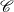be a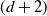-angulated category with d-suspension functor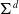. Our main results show that every Serre functor onis a-angulated functor. We also show thathas a Serre functor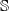if and only ifhas Auslander–Reiten-angles. Moreover,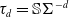where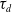is d-Auslander–Reiten translation. These results generalize work by Bondal–Kapranov and Reiten–Van den Bergh. As an application, we prove that for a strongly functorially finite subcategory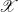of, the quotient category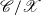is a-angulated category if and only if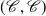is an-mutation pair, and if and only if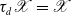.

]]>
https://dx.doi.org/10.1017/S0017089521000343?rft_dat=source%3Ddrssdoi:10.1017/S0017089521000343Higher-dimensional Auslander–Reiten theory on (d+2)-angulated categories52754710.1017/S0017089521000343https://dx.doi.org/10.1017/S0017089521000343?rft_dat=source%3Ddrss2021-10-27Glasgow Mathematical Journal2021-10-27Zhou, Panyue
<![CDATA[Cut cotorsion pairs]]>

We present the concept of cotorsion pairs cut along subcategories of an abelian category. This provides a generalization of complete cotorsion pairs, and represents a general framework to find approximations restricted to certain subcategories. We also exhibit some connections between cut cotorsion pairs and Auslander–Buchweitz approximation theory, by considering relative analogs for Frobenius pairs and Auslander–Buchweitz contexts. Several applications are given in the settings of relative Gorenstein homological algebra, chain complexes, and quasi-coherent sheaves, as well as to characterize some important results on the Finitistic Dimension Conjecture, the existence of right adjoints of quotient functors by Serre subcategories, and the description of cotorsion pairs in triangulated categories as co-t-structures.

]]>
https://dx.doi.org/10.1017/S0017089521000367?rft_dat=source%3Ddrssdoi:10.1017/S0017089521000367Cut cotorsion pairs54858510.1017/S0017089521000367https://dx.doi.org/10.1017/S0017089521000367?rft_dat=source%3Ddrss2021-12-10Glasgow Mathematical Journal2021-12-10Huerta, MindyMendoza, OctavioPérez, Marco A.
<![CDATA[A few remarks on Pimsner–Popa bases and regular subfactors of depth 2]]>

We prove that a finite index regular inclusion of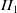-factors with commutative first relative commutant is always a crossed product subfactor with respect to a minimal action of a biconnected weak Kac algebra. Prior to this, we prove that every finite index inclusion of-factors which is of depth 2 and has simple first relative commutant (respectively, is regular and has commutative or simple first relative commutant) admits a two-sided Pimsner–Popa basis (respectively, a unitary orthonormal basis).

]]>
https://dx.doi.org/10.1017/S0017089521000379?rft_dat=source%3Ddrssdoi:10.1017/S0017089521000379A few remarks on Pimsner–Popa bases and regular subfactors of depth 258660210.1017/S0017089521000379https://dx.doi.org/10.1017/S0017089521000379?rft_dat=source%3Ddrss2021-12-01Glasgow Mathematical Journal2021-12-01Bakshi, Keshab ChandraGupta, Ved Prakash
<![CDATA[The Frucht property in the quantum group setting]]>

A classical theorem of Frucht states that any finite group appears as the automorphism group of a finite graph. In the quantum setting, the problem is to understand the structure of the compact quantum groups which can appear as quantum automorphism groups of finite graphs. We discuss here this question, notably with a number of negative results.

]]>
https://dx.doi.org/10.1017/S0017089521000380?rft_dat=source%3Ddrssdoi:10.1017/S0017089521000380The Frucht property in the quantum group setting60363310.1017/S0017089521000380https://dx.doi.org/10.1017/S0017089521000380?rft_dat=source%3Ddrss2021-11-29Glasgow Mathematical Journal2021-11-29Banica, T.McCarthy, J.P.
<![CDATA[Abelian actions on compact nonorientable Riemann surfaces]]>

Given an integer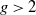, we state necessary and sufficient conditions for a finite Abelian group to act as a group of automorphisms of some compact nonorientable Riemann surface of genus g. This result provides a new method to obtain the symmetric cross-cap number of Abelian groups. We also compute the least symmetric cross-cap number of Abelian groups of a given order and solve the maximum order problem for Abelian groups acting on nonorientable Riemann surfaces.

]]>
https://dx.doi.org/10.1017/S0017089521000410?rft_dat=source%3Ddrssdoi:10.1017/S0017089521000410Abelian actions on compact nonorientable Riemann surfaces63464810.1017/S0017089521000410https://dx.doi.org/10.1017/S0017089521000410?rft_dat=source%3Ddrss2021-12-02Glasgow Mathematical Journal2021-12-02Rodríguez, Jesús
<![CDATA[Derived categories of skew-gentle algebras and orbifolds]]>

Skew-gentle algebras are a generalisation of the well-known class of gentle algebras with which they share many common properties. In this work, using non-commutative Gröbner basis theory, we show that these algebras are strong Koszul and that the Koszul dual is again skew-gentle. We give a geometric model of their bounded derived categories in terms of polygonal dissections of surfaces with orbifold points, establishing a correspondence between curves in the orbifold and indecomposable objects. Moreover, we show that the orbifold dissections encode homological properties of skew-gentle algebras such as their singularity categories, their Gorenstein dimensions and derived invariants such as the determinant of their q-Cartan matrices.

]]>
https://dx.doi.org/10.1017/S0017089521000422?rft_dat=source%3Ddrssdoi:10.1017/S0017089521000422Derived categories of skew-gentle algebras and orbifolds64967410.1017/S0017089521000422https://dx.doi.org/10.1017/S0017089521000422?rft_dat=source%3Ddrss2022-01-31Glasgow Mathematical Journal2022-01-31Labardini-Fragoso, DanielSchroll, SibylleValdivieso, Yadira
<![CDATA[Principal bundles on two-dimensional CW-complexes with disconnected structure group]]>

Given any topological group G, the topological classification of principal G-bundles over a finite CW-complex X is long known to be given by the set of free homotopy classes of maps from X to the corresponding classifying space BG. This classical result has been long-used to provide such classification in terms of explicit characteristic classes. However, even when X has dimension 2, there is a case in which such explicit classification has not been explicitly considered. This is the case where G is a Lie group, whose group of components acts nontrivially on its fundamental group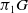. Here, we deal with this case and obtain the classification, in terms of characteristic classes, of principal G-bundles over a finite CW-complex of dimension 2, with G is a Lie group such that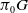is abelian.

]]>
https://dx.doi.org/10.1017/S0017089521000434?rft_dat=source%3Ddrssdoi:10.1017/S0017089521000434Principal bundles on two-dimensional CW-complexes with disconnected structure group67569010.1017/S0017089521000434https://dx.doi.org/10.1017/S0017089521000434?rft_dat=source%3Ddrss2022-01-06Glasgow Mathematical Journal2022-01-06Oliveira, André G.
<![CDATA[Hausdorff dimension of the set of almost convergent sequences]]>

The paper deals with the sets of numbers from [0,1] such that their binary representation is almost convergent. The aim of the study is to compute the Hausdorff dimensions of such sets. Previously, the results of this type were proved for a single summation method (e.g. Cesàro, Abel, Toeplitz). This study extends the results to a wide range of matrix summation methods.

]]>
https://dx.doi.org/10.1017/S0017089521000446?rft_dat=source%3Ddrssdoi:10.1017/S0017089521000446Hausdorff dimension of the set of almost convergent sequences69169710.1017/S0017089521000446https://dx.doi.org/10.1017/S0017089521000446?rft_dat=source%3Ddrss2022-01-06Glasgow Mathematical Journal2022-01-06Usachev, Alexandr
<![CDATA[Weak amenability of free products of hyperbolic and amenable groups]]>

We show that if G is an amenable group and H is a hyperbolic group, then the free product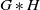is weakly amenable. A key ingredient in the proof is the fact that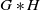is orbit equivalent to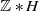.

]]>
https://dx.doi.org/10.1017/S0017089521000458?rft_dat=source%3Ddrssdoi:10.1017/S0017089521000458Weak amenability of free products of hyperbolic and amenable groups69870110.1017/S0017089521000458https://dx.doi.org/10.1017/S0017089521000458?rft_dat=source%3Ddrss2022-01-06Glasgow Mathematical Journal2022-01-06Vergara, Ignacio
<![CDATA[Proper Lie automorphisms of incidence algebras]]>

Let X be a finite connected poset and K a field. We study the question, when all Lie automorphisms of the incidence algebra I(X, K) are proper. Without any restriction on the length of X, we find only a sufficient condition involving certain equivalence relation on the set of maximal chains of X. For some classes of posets of length one, such as finite connected crownless posets (i.e., without weak crown subposets), crowns, and ordinal sums of two anti-chains, we give a complete answer.

]]>
https://dx.doi.org/10.1017/S0017089522000015?rft_dat=source%3Ddrssdoi:10.1017/S0017089522000015Proper Lie automorphisms of incidence algebras70271510.1017/S0017089522000015https://dx.doi.org/10.1017/S0017089522000015?rft_dat=source%3Ddrss2022-02-07Glasgow Mathematical Journal2022-02-07Fornaroli, Érica Z.Khrypchenko, MykolaSantulo, Ednei A.
<![CDATA[The nonclassical diffusion equations with time-dependent memory kernels and a new class of nonlinearities]]>

In this study, we consider the nonclassical diffusion equations with time-dependent memory kernels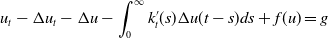on a bounded domain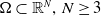. Firstly, we study the existence and uniqueness of weak solutions and then, we investigate the existence of the time-dependent global attractors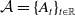in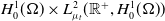. Finally, we prove that the asymptotic dynamics of our problem, when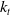approaches a multiple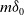of the Dirac mass at zero as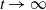, is close to the one of its formal limit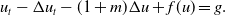The main novelty of our results is that no restriction on the upper growth of the nonlinearity is imposed and the memory kernel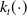depends on time, which allows for instance to describe the dynamics of aging materials.

]]>
https://dx.doi.org/10.1017/S0017089522000027?rft_dat=source%3Ddrssdoi:10.1017/S0017089522000027The nonclassical diffusion equations with time-dependent memory kernels and a new class of nonlinearities71673310.1017/S0017089522000027https://dx.doi.org/10.1017/S0017089522000027?rft_dat=source%3Ddrss2022-02-21Glasgow Mathematical Journal2022-02-21Thuy, Le ThiToan, Nguyen Duong
<![CDATA[Property (T) in k-gonal random groups]]>

The k-gonal models of random groups are defined as the quotients of free groups on n generators by cyclically reduced words of length k. As k tends to infinity, this model approaches the Gromov density model. In this paper, we show that for any fixed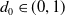, if positive k-gonal random groups satisfy Property (T) with overwhelming probability for densities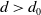, then so do jk-gonal random groups, for any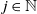. In particular, this shows that for densities above 1/3, groups in 3k-gonal models satisfy Property (T) with probability 1 as n approaches infinity.

]]>
https://dx.doi.org/10.1017/S0017089522000040?rft_dat=source%3Ddrssdoi:10.1017/S0017089522000040Property (T) in k-gonal random groups73473810.1017/S0017089522000040https://dx.doi.org/10.1017/S0017089522000040?rft_dat=source%3Ddrss2022-02-22Glasgow Mathematical Journal2022-02-22Montee, MurphyKate
<![CDATA[A note on holomorphic sectional curvature of a hermitian manifold]]>

As is well known, the holomorphic sectional curvature is just half of the sectional curvature in a holomorphic plane section on a Kähler manifold (Zheng, Complex differential geometry (2000)). In this article, we prove that if the holomorphic sectional curvature is half of the sectional curvature in a holomorphic plane section on a Hermitian manifold then the Hermitian metric is Kähler.

]]>
https://dx.doi.org/10.1017/S0017089522000064?rft_dat=source%3Ddrssdoi:10.1017/S0017089522000064A note on holomorphic sectional curvature of a hermitian manifold73974510.1017/S0017089522000064https://dx.doi.org/10.1017/S0017089522000064?rft_dat=source%3Ddrss2022-03-04Glasgow Mathematical Journal2022-03-04Li, HongjunQiu, Chunhui
<![CDATA[Homotopy commutativity in Hermitian symmetric spaces]]>

Ganea proved that the loop space of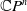is homotopy commutative if and only if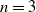. We generalize this result to that the loop spaces of all irreducible Hermitian symmetric spaces but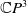are not homotopy commutative. The computation also applies to determining the homotopy nilpotency class of the loop spaces of generalized flag manifolds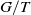for a maximal torus T of a compact, connected Lie group G.

]]>
https://dx.doi.org/10.1017/S0017089522000118?rft_dat=source%3Ddrssdoi:10.1017/S0017089522000118Homotopy commutativity in Hermitian symmetric spaces74675210.1017/S0017089522000118https://dx.doi.org/10.1017/S0017089522000118?rft_dat=source%3Ddrss2022-04-18Glasgow Mathematical Journal2022-04-18Kishimoto, DaisukeTakeda, MasahiroTong, Yichen
<![CDATA[GMJ volume 64 issue 3 Cover and Front matter]]>https://dx.doi.org/10.1017/S001708952200026X?rft_dat=source%3Ddrssdoi:10.1017/S001708952200026XGMJ volume 64 issue 3 Cover and Front matter1210.1017/S001708952200026Xhttps://dx.doi.org/10.1017/S001708952200026X?rft_dat=source%3Ddrss2022-08-05Glasgow Mathematical Journal2022-08-05<![CDATA[GMJ volume 64 issue 3 Cover and Back matter]]>https://dx.doi.org/10.1017/S0017089522000271?rft_dat=source%3Ddrssdoi:10.1017/S0017089522000271GMJ volume 64 issue 3 Cover and Back matter1310.1017/S0017089522000271https://dx.doi.org/10.1017/S0017089522000271?rft_dat=source%3Ddrss2022-08-05Glasgow Mathematical Journal2022-08-05